Math Olympiad Test: Heron’s Formula- 2

# Math Olympiad Test: Heron’s Formula- 2

Test Description

## 15 Questions MCQ Test Mathematics Olympiad for Class 9 | Math Olympiad Test: Heron’s Formula- 2

Math Olympiad Test: Heron’s Formula- 2 for Class 9 2022 is part of Mathematics Olympiad for Class 9 preparation. The Math Olympiad Test: Heron’s Formula- 2 questions and answers have been prepared according to the Class 9 exam syllabus.The Math Olympiad Test: Heron’s Formula- 2 MCQs are made for Class 9 2022 Exam. Find important definitions, questions, notes, meanings, examples, exercises, MCQs and online tests for Math Olympiad Test: Heron’s Formula- 2 below.
Solutions of Math Olympiad Test: Heron’s Formula- 2 questions in English are available as part of our Mathematics Olympiad for Class 9 for Class 9 & Math Olympiad Test: Heron’s Formula- 2 solutions in Hindi for Mathematics Olympiad for Class 9 course. Download more important topics, notes, lectures and mock test series for Class 9 Exam by signing up for free. Attempt Math Olympiad Test: Heron’s Formula- 2 | 15 questions in 15 minutes | Mock test for Class 9 preparation | Free important questions MCQ to study Mathematics Olympiad for Class 9 for Class 9 Exam | Download free PDF with solutions
 1 Crore+ students have signed up on EduRev. Have you?
Math Olympiad Test: Heron’s Formula- 2 - Question 1

### The area of an isosceles triangles whose equal sides are 12cm and the other side is 6cm long, will be:

Detailed Solution for Math Olympiad Test: Heron’s Formula- 2 - Question 1

In ∆ABD,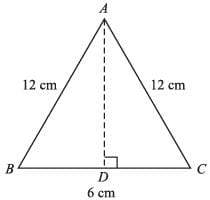AB2 + BD2 = AD2
⇒ AD2 = AB2 – BD2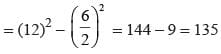⇒ AD = √135 = 3√15 cm
∴ area (∆ABC) = 1/2 x 6 x 3√15
= 9√15 cm2

Math Olympiad Test: Heron’s Formula- 2 - Question 2

### The sides of a triangle are 50 cm, 78 cm and 112 cm. the smallest altitude is:

Detailed Solution for Math Olympiad Test: Heron’s Formula- 2 - Question 2

Here S =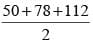= 120 cm
∴ Area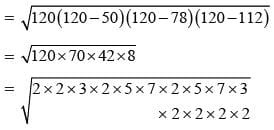= 2 × 3 × 2 × 2 × 2 × 5 × 7
= 240 × 7 cm2 = 1680 cm2
Area = 1/2 x base altitude = 1680 cm2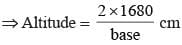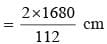= 30 cm

Math Olympiad Test: Heron’s Formula- 2 - Question 3

### The base and hypotenuse of a right triangle are 5 cm, 13 cm long. The length of altitude from the vertex containing right angle to the hypotenuse will be:

Detailed Solution for Math Olympiad Test: Heron’s Formula- 2 - Question 3

BC =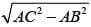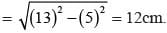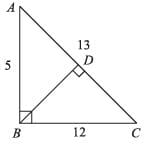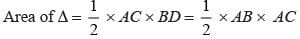⇒ AC × BD = AB × AC
⇒  13 × BD = 5 × 12
⇒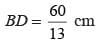Math Olympiad Test: Heron’s Formula- 2 - Question 4

Find the area of the given figure: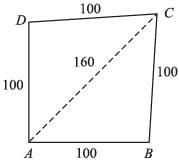Detailed Solution for Math Olympiad Test: Heron’s Formula- 2 - Question 4

In ∆DOC,
OD2 + OC2 = DC2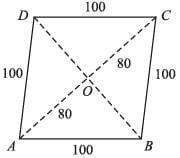⇒ OD =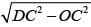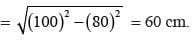∴ DB = 2 × OD = 2 × 60 = 120 cm.
Area of rhombus =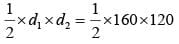= 9600 cm2

Math Olympiad Test: Heron’s Formula- 2 - Question 5

If a square and rhombus have same perimeter, and area of square is S and area of rhombus is R, then

Detailed Solution for Math Olympiad Test: Heron’s Formula- 2 - Question 5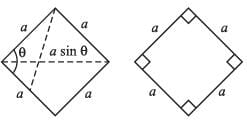Area of square = a2
Area of rhombus = a2 sin θ
∵ sin θ < 1
∴ a2 > a2 sin θ
ar(square) > ar(rhombus)
⇒ S > R

Math Olympiad Test: Heron’s Formula- 2 - Question 6

The area of kite in the adjoining figure is: (AC = BD = 32 cm)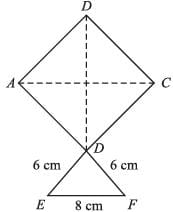Detailed Solution for Math Olympiad Test: Heron’s Formula- 2 - Question 6

Area of square ABCD = 1/2 × AC × BD
= 1/2 x 32 x 32
= 16 × 32 cm2
= 512 cm2
Here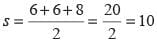Math Olympiad Test: Heron’s Formula- 2 - Question 7

Area of parallelogram, in the adjoining figure will be: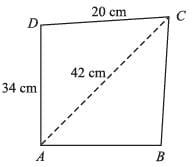Detailed Solution for Math Olympiad Test: Heron’s Formula- 2 - Question 7

Area of ∆ACD = area of ∆ACB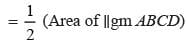⇒ area of ∆ACD =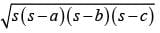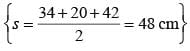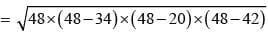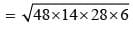= 4 × 6 × 7 × 2
= 4 × 84 = 336 cm2
∴ area of ∥gm = 2 × ar(∆ACD)
= 2 × 336 cm2
= 672 cm2

Math Olympiad Test: Heron’s Formula- 2 - Question 8

Find the area of the shaded region.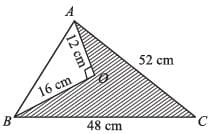Detailed Solution for Math Olympiad Test: Heron’s Formula- 2 - Question 8

In ∆AOB,
AB =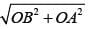= 20 cm.
∴ ar(∆AOB) = 1/2 × 12 × 16 = 96 cm2
For ∆ABC,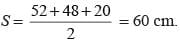∴ ar (∆ABC) =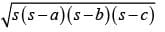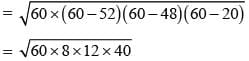= 480 cm2
∴ Area of shaded region
= 480 – 96
= 384 cm2

Math Olympiad Test: Heron’s Formula- 2 - Question 9

A square and an equilateral triangle have equal perimeters. If the diagonal of the square is 12√2 cm, the area of the triangle is:

Detailed Solution for Math Olympiad Test: Heron’s Formula- 2 - Question 9

Length of equilateral triangle = x/3 cm
Length of side of square = x/4 cm
Length of side of square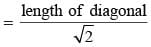= 12 cm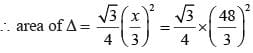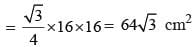Math Olympiad Test: Heron’s Formula- 2 - Question 10

The third side of triangle whose two sides are 26 and 28 cm and area is 336 cm2, is

Detailed Solution for Math Olympiad Test: Heron’s Formula- 2 - Question 10

Let the length of third side be x
∴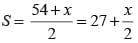Area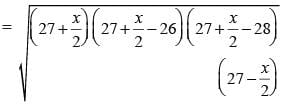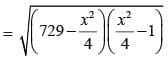⇒ x = 30 cm
∴ Third side = 30 cm

Math Olympiad Test: Heron’s Formula- 2 - Question 11

The length of sides of a triangle are in the ratio 3 : 4 : 5 and its perimeter is 144 cm, then, the height corresponding to the length side is:

Detailed Solution for Math Olympiad Test: Heron’s Formula- 2 - Question 11

Lengths of sides of triangle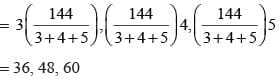∴ Area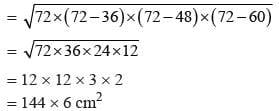∴ Height corresponding to the longest side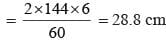Math Olympiad Test: Heron’s Formula- 2 - Question 12

ABCD is a parallelogram, where,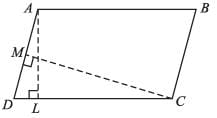AL = 8 cm, CM = 10 cm, AD = 6 cm. Find AB.

Detailed Solution for Math Olympiad Test: Heron’s Formula- 2 - Question 12

Area of ∥gm = AL × DC = CM × AD
⇒ 8 × DC = 10 × 6
⇒ DC = 60/8 = 7.5 cm = AB.

Math Olympiad Test: Heron’s Formula- 2 - Question 13

The area of the adjoining figure will be: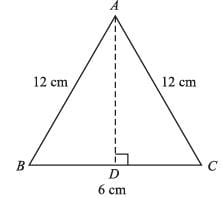Detailed Solution for Math Olympiad Test: Heron’s Formula- 2 - Question 13

Here S =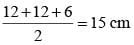∴ Required area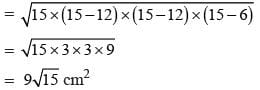Math Olympiad Test: Heron’s Formula- 2 - Question 14

If each side of ∆ is doubled, then the area will become how many times?

Detailed Solution for Math Olympiad Test: Heron’s Formula- 2 - Question 14

If the sides of ∆ are a, b and c
∴ New sides are 2a, 2b, 2c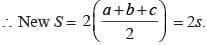∴ New area =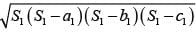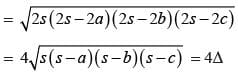Math Olympiad Test: Heron’s Formula- 2 - Question 15

The diagonal of a parallelogram divide it in 2 parts, the area of the two parts:

Detailed Solution for Math Olympiad Test: Heron’s Formula- 2 - Question 15

The two parts of the parallelogram are congruent because they have equal area.

## Mathematics Olympiad for Class 9

1 videos|43 tests
 Use Code STAYHOME200 and get INR 200 additional OFF Use Coupon Code
Information about Math Olympiad Test: Heron’s Formula- 2 Page
In this test you can find the Exam questions for Math Olympiad Test: Heron’s Formula- 2 solved & explained in the simplest way possible. Besides giving Questions and answers for Math Olympiad Test: Heron’s Formula- 2, EduRev gives you an ample number of Online tests for practice

## Mathematics Olympiad for Class 9

1 videos|43 tests